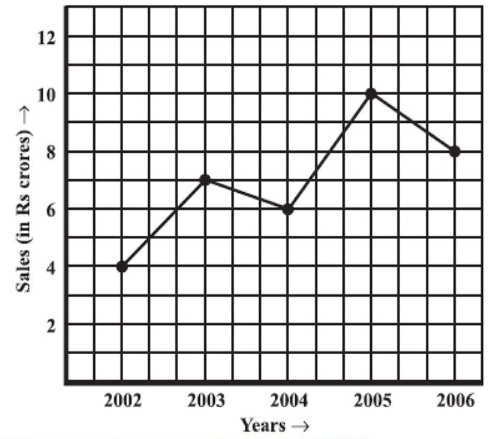# Ex.15.1 Q2 Introduction to Graphs Solutions - NCERT Maths Class 8

Go back to  'Ex.15.1'

## Question

The following line graph shows the yearly sales figure for a manufacturing company.

(a) What were the sales in (i) $$2002$$ (ii) $$2006$$?

(b) What were the sales in (i) $$2003$$ (ii) $$2005$$?

(c) Compute the difference between the sales in $$2002$$ and $$2006.$$

(d) In which year was there the greatest difference between the sales as compared to its previous year?## Text Solution

Reasoning:

Horizontal axis represents ‘Years’ and vertical represents ‘Sales’ in Rs crore.

We can find the value of ‘Sales’ on vertical axis according to the given value of ‘Years’ on the horizontal axis value or vice versa.

Steps:

(a)

(i) In $$2002,$$ the sales were $$\rm{Rs}\; 4$$ crores.

(ii) In $$2006,$$ the sales were $$\rm{Rs}\; 8$$ crores.

(b)

(i) In $$2003,$$ the sales were $$\rm{Rs}\; 7$$ crores.

(ii) In $$2005,$$ the sales were $$\rm{Rs}\; 10$$ crores.

(c)

(i) In $$2002,$$ the sales were $$\rm{Rs}\; 4$$ crores and in $$2006,$$ the sales were $$\rm{Rs}\; 8$$ crores.

Difference between the sales in $$2002$$ and $$2006$$

$$= \rm{Rs} \;(8 − 4)$$ crores $$=$$ $$\rm{Rs}\; 4$$ crores

(d) Difference between the sales of the year $$2006$$ and $$2005$$

$$= \rm{Rs}\; (10 − 8)$$ crores $$=\rm{ Rs}\; 2$$ crores

Difference between the sales of the year $$2005$$ and $$2004$$

$$= \rm{Rs}\; (10 − 6)$$ crores $$=\rm{Rs}\; 4$$ crores

Difference between the sales of the year $$2004$$ and $$2003$$

$$=\rm{Rs}\; (7 − 6)$$ crore $$= \rm{Rs}\; 1$$ crore

Difference between the sales of the year $$2003$$ and $$2002$$

$$= \rm{Rs} \;(7 − 4)$$ crores $$=\rm{Rs}\; 3$$ crores

Hence, the difference was the maximum in the year $$2005$$ as compared to its previous year $$2004.$$

Learn from the best math teachers and top your exams

• Live one on one classroom and doubt clearing
• Practice worksheets in and after class for conceptual clarity
• Personalized curriculum to keep up with school